# My语言几种常见指标编写与跨指标模型的案例分析

Author: , Created: 2019-07-08 10:01:48, Updated: 2019-07-16 15:38:53

My语言不止适用于国内商品期货，对于数字货币相关交易也是无功能差别的同样支持。

### 几个常用的指标分类与编写

• 传统经典的K线形态包括：三足乌鸦，十字星，穿头破脚，一柱擎天，仙人指路，金针探底等等

• 指标方面，其中趋势类指标又分为：MA(均线组合），BOLL，PUBU(瀑布线），SAR（止损点）等等

• 摆动类指标：ATR（真实波幅）、KDJ（随机指标）、MACD、WR（威廉指标）等等

• 量仓分析指标 CJL（成交量）、DUALVOL（多空量比）、OBV（量能潮）

``````AA:=OPEN=LOW;
BB:=CLOSE=HIGH;
CC :=CLOSE/OPEN>1.04;
``````

``````A1:=REF(CLOSE,1);
A2:=REF(OPEN,1);
BB:C/O>1.04;
B1:=OPEN<A1;
B2:=CLOSE>A2;
``````

• 均线交叉：5日均线向上穿过10日均线（金叉）；5日均线向下穿过10日均线（死叉）
``````MA5:=MA(CLOSE,5);
MA10:=MA(CLOSE,10);

``````

``````MA5:=MA(CLOSE,5);
MA10:=MA(CLOSE,10);
MA30:=MA(CLOSE,30);
CC:=MA5>MA30 AND MA10>MA30；
``````
• BOLL通道： BOLL由下轨(BOTTOM)、上轨(TOP)、中线(MID)三者组成通道。通道变宽并向上时，价格在上轨之上，多头趋势来临。反之空头趋势来临。

``````MID:MA(CLOSE,26);//求N个周期的收盘价均线，称为布林通道中轨
TMP2:=STD(CLOSE,26);//求M个周期内的收盘价的标准差
TOP:MID+2*TMP2;//布林通道上轨
BOTTOM:MID-2*TMP2;//布林通道下轨
AA:=TOP>REF(TOP,1)&&BOTTOM<REF(BOTTOM,1)&&MID>REF(MID,1);
BB:=C>TOP;
``````

• SAR（止损点）指标：SAR向上穿过0，多头趋势；SAR向下穿过0，空头趋势。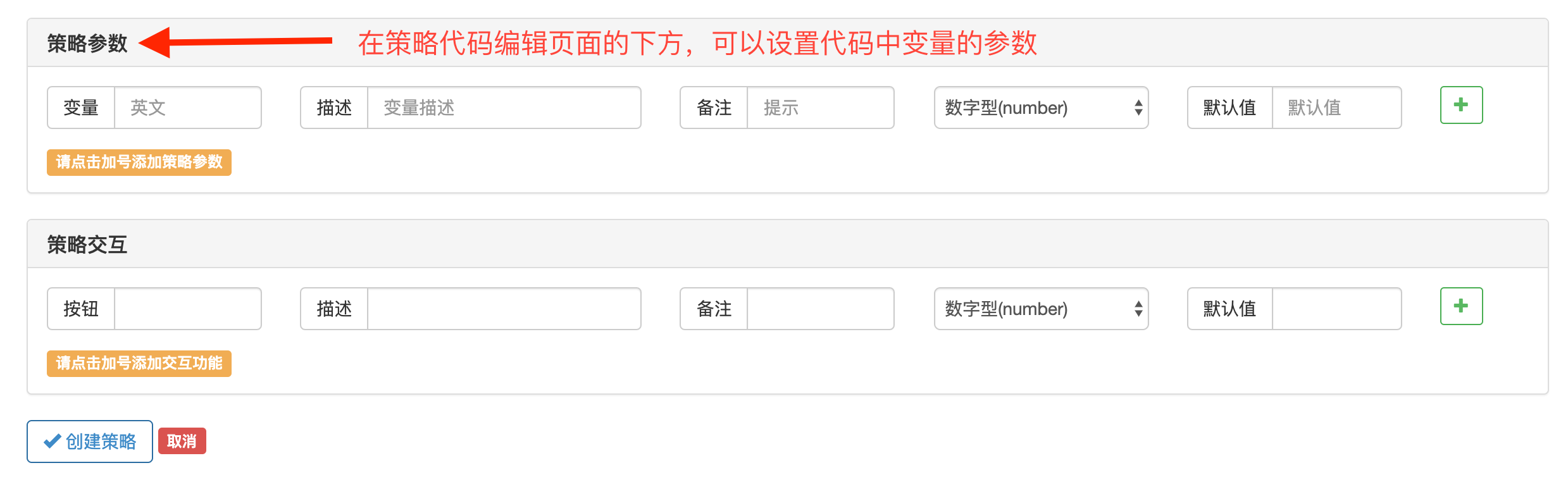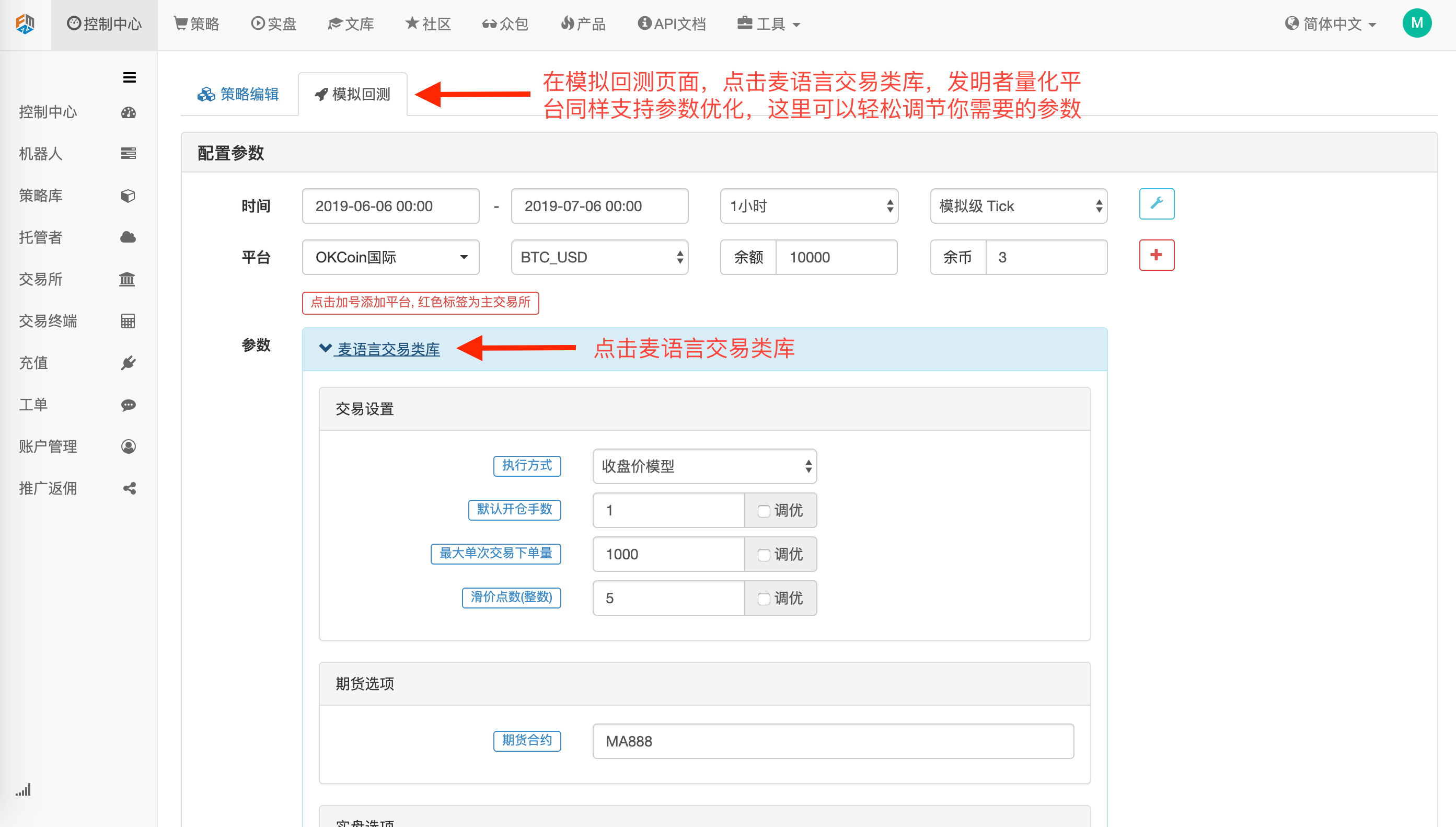N 1 100 4 STEP 1 20 2 MVALUE 1 10 2

``````STEP1:=STEP/100;
MVALUE1:=MVALUE/10;
SARLINE:SAR(N,STEP1,MVALUE1),CIRCLEDOT;
//N个周期的抛物转向，步长为STEP1，极限值为MVALUE1.
CROSS(SARLINE,0),BPK;//抛物转向值上穿0，做多。
CROSS(0,SARLINE),SPK;//抛物转向值下穿0，作空。
AUTOFILTER;
``````

• KDJ随机指标由K、D、J三条线组成，指标线介于0~100之间变化，J线低于20，进入超卖区，J线高于80，进入超买区。如果K线上穿D线可以做多，如果K线下穿D线可以做空。
``````RSV:=(CLOSE-LLV(LOW,N))/(HHV(HIGH,N)-LLV(LOW,N))*100;
//收盘价与N周期最低值做差，N周期最高值与N周期最低值做差，两差之间做比值。
K:SMA(RSV,M1,1);//RSV的移动平均值
D:SMA(K,M2,1);//K的移动平均值
J:3*K-2*D;
BACKGROUNDSTYLE(1);
CROSS(K,D),BPK;//KD金叉，做多。
CROSS(J,20),BP;//J值上穿20
CROSS(D,K),SPK;//KD死叉，做空。
CROSS(80,J),SP;// J值下穿80
AUTOFILTER;
``````
• MACD指数平滑异同平均线，是从双指数移动平均线发展而来的，由快的指数移动平均线（EMA）减去慢的指数移动平均线，MACD的意义和双移动平均线基本相同，当MACD从负数转向正数，是做多信号。当MACD从正数转向负数，是做空信号。
``````DIFF:EMA(CLOSE,12) - EMA(CLOSE,26);
//短周期与长周期的收盘价的指数平滑移动平均值做差。
DEA:EMA(DIFF,9);//DIFF的M个周期指数平滑移动平均
2*(DIFF-DEA),COLORSTICK;//DIFF减DEA的2倍画柱状线
CROSS(DIFF,DEA),BPK;//DIFF上穿DEA,做多。
CROSS(DEA,DIFF),SPK;//DIFF下穿DEA,做空。
AUTOFILTER;
``````

### 跨指标模型案例

• 第一个原因：模型中跨指标，是技术面内部要素共振的一种交易思想，将多个不同分类指标交易思想结合在一起进行看盘断势。

• 第二个原因：趋势类指标在盘整行情中失效，单独使用摆动类指标无法判断当前行情状态，需要多个指标结合分析。

• 趋势判断与精细分析相结合。
• 多条件同向判断。

### 震荡模型的例子

• 潮汐指数
``````ABS(CLOSE-REF(CLOSE,29))/(HHV(HIGH,30)-LLV(LOW,30))*100
``````

``````RSV:=(CLOSE-LLV(LOW,N))/(HHV(HIGH,N)-LLV(LOW,N))*100;
K:SMA(RSV,M1,1);//RSV的移动平均值
D:SMA(K,M2,1);//K的移动平均值
J:3*K-2*D;
CMIVAL:ABS(CLOSE-REF(CLOSE,29))/(HHV(HIGH,30)-LLV(LOW,30))*100,NODRAW;
BACKGROUNDSTYLE(1);
K>D&&EVERY(CMIVAL<20,2),BPK;//盘整行情，KD金叉，做多。
CROSS(J,10)||CROSS(K,D),BP;// J值上穿10超卖或者KD金叉，平仓
D>K&&EVERY(CMIVAL<20,2),SPK;//盘整行情，KD死叉，做空。
CROSS(90,J)||CROSS(D,K),SP;// J值下穿90超买或者KD死叉，平仓
AUTOFILTER;
``````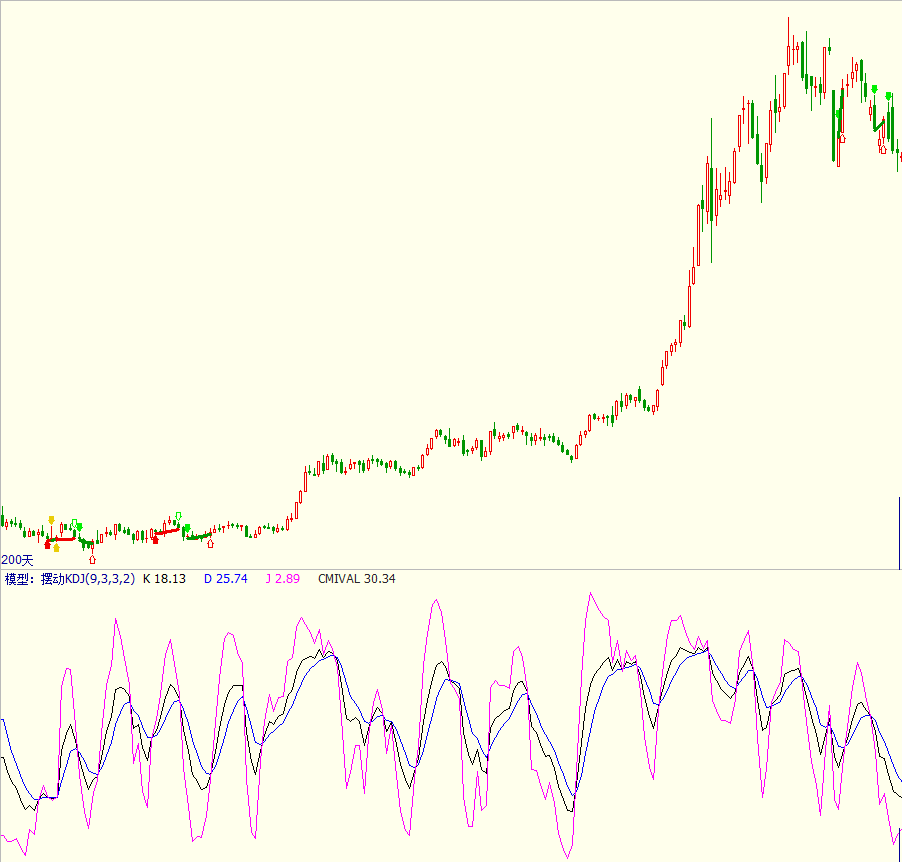### 趋势模型的例子

EMA趋势判断交易系统：

``````UPPERMA:EMA(HIGH,30);//计算30根K线最高价的EMA
LOWERMA:EMA(LOW,30);//计算30根K线最低价的EMA
CROSSUP(C,UPPERMA),BPK;//收盘价上穿EMA，做多
CROSSDOWN(C,LOWERMA),SPK;//收盘价下穿EMA，做空
AUTOFILTER;
``````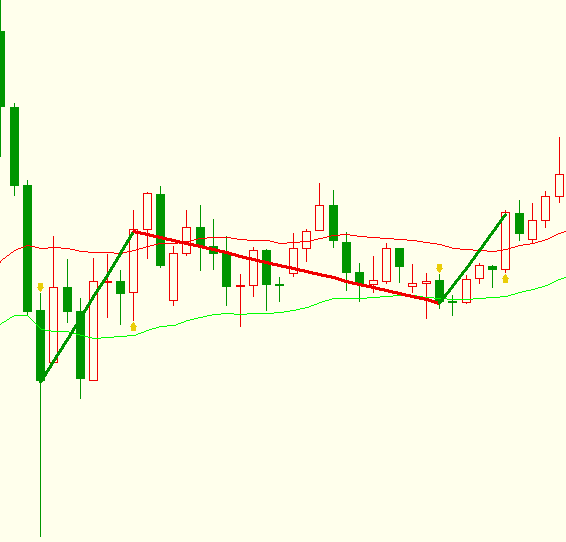``````TR:=SUM(MAX(MAX(HIGH-LOW,ABS(HIGH-REF(CLOSE,1))),ABS(LOW-REF(CLOSE,1))),14);
HD:=HIGH-REF(HIGH,1);//最高价与前一周期最高价做差
LD:=REF(LOW,1)-LOW;//前一周期最低价与最低价做差
DMP:=SUM(IFELSE(HD>0 && HD>LD,HD,0),14);
//如果HD>0并且HD>LD,取HD否则取0,对取值做N周期累加求和。
DMM:=SUM(IFELSE(LD>0 && LD>HD,LD,0),14);
//如果LD>0并且LD>HD,取LD否则取0,对取值做N周期累加求和。
PDI:=DMP*100/TR;
MDI:=DMM*100/TR;
``````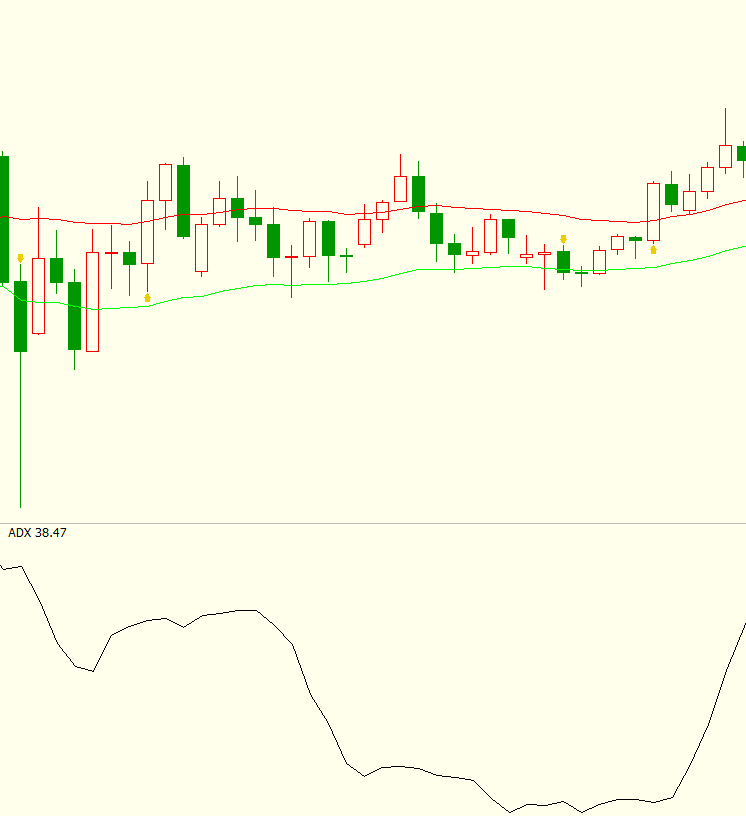``````TR:=SUM(MAX(MAX(HIGH-LOW,ABS(HIGH-REF(CLOSE,1))),ABS(LOW-REF(CLOSE,1))),14);
HD:=HIGH-REF(HIGH,1);
LD:=REF(LOW,1)-LOW;
DMP:=SUM(IFELSE(HD>0 && HD>LD,HD,0),14);
DMM:=SUM(IFELSE(LD>0 && LD>HD,LD,0),14);
PDI:=DMP*100/TR;
MDI:=DMM*100/TR;
UPPERMA:=EMA(HIGH,30);
LOWERMA:=EMA(LOW,30);
AUTOFILTER;
``````

### 多个同类指标同向判断 以精确分析，提高胜率

• 昨日价格大于布林通道上轨，并且当日周期价格大于唐奇安通道上轨，开多单

• 昨日价格小于布林通道下轨，并且当日周期价格小于唐奇安通道下轨，开空单

• 持有多单时，价格小于自适应出场均线，平多单

• 持有空单时，价格大于自适应出场均线，平空单

``````//当日市场波动
TODAYVOLATILITY:=STD(CLOSE,30);
//昨日市场波动
YESTERDAYVOLATILITY:=REF(TODAYVOLATILITY,1);
//市场波动的变动率
DELTAVOLATILITY:(TODAYVOLATILITY-YESTERDAYVOLATILITY)/TODAYVOLATILITY;
//计算自适应参数
LOOKBACKDAYS1:=LOOP2(BARPOS=30,20,REF(LOOKBACKDAYS1,1)*(1+DELTAVOLATILITY));
LOOKBACKDAYS2:=ROUND(LOOKBACKDAYS1,0);
LOOKBACKDAYS3:=MIN(LOOKBACKDAYS2,60);//60自适应参数的上限
LOOKBACKDAYS:=MAX(LOOKBACKDAYS3,20);//20自适应参数的下限
//自适应布林通道中轨
MIDLINE:=MA(CLOSE,LOOKBACKDAYS);
BAND:=STD(CLOSE,LOOKBACKDAYS);
//自适应布林通道上轨
UPBAND:=MIDLINE+2*BAND;
//自适应布林通道下轨
DNBAND:=MIDLINE-2*BAND;
//自适应唐奇安通道上轨
//自适应唐奇安通道下轨
SELLPOINT:=LLV(LOW,LOOKBACKDAYS);
//自适应出场均线
LIQPOINT:=MIDLINE;
//昨日价格大于布林通道上轨，并且当日价格大于唐奇安通道上轨，开多单
//持有多单时，昨日价格小于布林通道下轨，并且当日价格小于唐奇安通道下轨，平多单
REF(C,1)<REF(DNBAND,1)&&LOW<=REF(SELLPOINT,1),SP;
//持有多单时，价格小于自适应出场均线，平多单
BARSBK>=1&&LOW<=REF(LIQPOINT,1),SP;
//持有空单时，昨日价格大于布林通道上轨，并且当日价格大于唐奇安通道上轨，平空单
//昨日价格小于布林通道下轨，并且当日价格小于唐奇安通道下轨，开空单
REF(CLOSE,1)<REF(DNBAND,1)&&LOW<=REF(SELLPOINT,1),SK;
//持有空单时，价格大于自适应出场均线，平空单